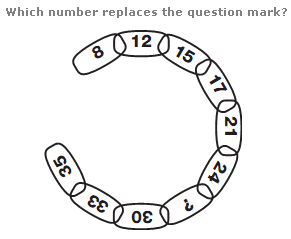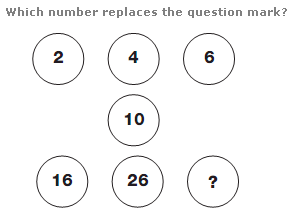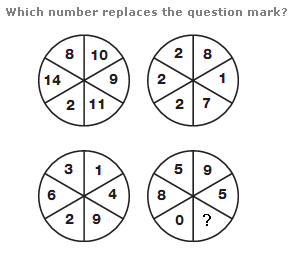# Puzzles - Number puzzles

### Exercise :: Number puzzlesAnswer : 26 Explanation : Moving clockwise around the chain, numbers increase by 4, then 3, then 2, before repeating this pattern.Answer : 42 Explanation : Starting in the top left and moving in a Z shape around the diagram, each number equals the sum of the previous 2 numbers.Answer : 2 Explanation : The numbers in the segments of the top left circle equal the sum of the numbers in corresponding segments of the lower two circles, and the numbers in the top right circle equal the difference between numbers in corresponding segments of the lower two circles.